• matlab加入单频噪声并滤波 加入白噪声并滤波网络互联
• ## MATLAB手写数字识别

万次阅读 多人点赞 2019-07-31 14:07:22
本文主要是根据《matlab手写神经网络实现识别手写数字》博客中的代码进行试验。由于没有数据集，所以采用了MNIST数据集进行代码的运行。数据集不同所以需要对代码进行微小改动。 简介 数据处理：4000张作为训练样本...
本文主要是根据《matlab手写神经网络实现识别手写数字》博客中的代码进行试验。由于没有数据集，所以采用了MNIST数据集进行代码的运行。数据集不同所以需要对代码进行微小改动。
简介
数据处理：4000张作为训练样本，1000张作为测试样本； 图像大小：图片的灰度值矩阵（28，28）； 图像名称：由标签和顺序号组成。标签_顺序号.bmp 训练样本：每个数字的图像名称的顺序号是从0-399，各400个。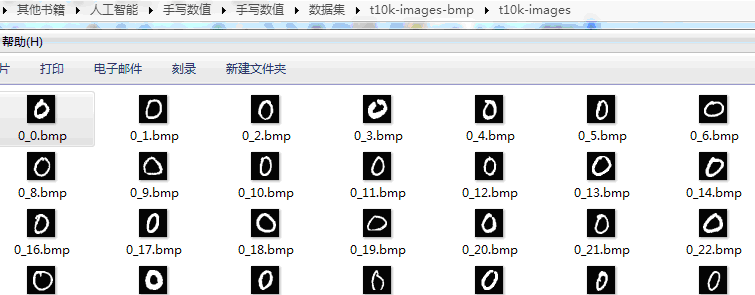测试样本：每个数字的图像名称的顺序号是从401-500，各100个。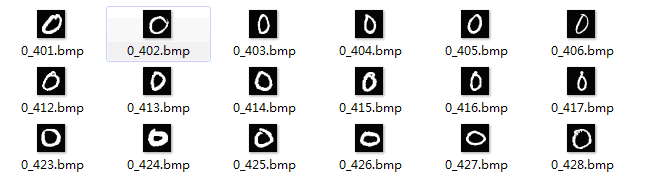标签制作
标签格式： 训练数据集中前400个标签都是[1,0,0,0,0,0,0,0,0,0]代表数字0，401-800个标签都是[0,1,0,0,0,0,0,0,0,0]代表数字2，其余类推。这也就是所谓的onehot 由于数据集不同，图像的格式也不一样等因素，需要对代码稍微做修改，具体如下： 制作label时遇到的障碍，xlswrite()函数在写入矩阵时对矩阵大小有限制，一定要小心，因为我的电脑安装的是2003，所以无法对4000列数据直接写入，只好行列互换后再存储，代码将生成两个xsl文件，分别是label.xsl和label2.xsl,分别是训练数据和测试数据的标签。 增加一个label_create.m文件，用来新建标签文件。 label_create.m代码：
% 创建Excel存储label
% 根据图片的名字,例如：3_101.bmp，第一个数值是标签，第二个是图片数。
% 为了转换成神经网络的标签，0-9这10个标签应转换为[1,0,0,0,0,0,0,0,0,0],[0,1,0,0,0,0,0,0,0,0]
% 这样的格式，即第几位是1就代表标签是几？如[0,1,0,0,0,0,0,0,0,0]代表2
% 例如我们的前 400个图都是0，所以标签都是[1,0,0,0,0,0,0,0,0,0]
% 因为每个版本的Excel允许存储的行列不同，这里是2003版存储不下4000列，故需要将label矩阵转置后才能存下
% Excel2003可存储：65536*256
clear all;
clc;
label=zeros(10,4000);
label2=zeros(10,1000);

eye_sam=eye(10);
for j=1:10
label(:,(400*(j-1)+1):400*j)= repmat( eye_sam(:,j),1,400 );
end
T=label';
xlswrite('D:\Documents\MATLAB\label.xls',T);

for j=1:10
label2(:,(100*(j-1)+1):100*j)= repmat( eye_sam(:,j),1,100 );
end
T2=label2';
xlswrite('D:\Documents\MATLAB\label2.xls',T2);

getdata.m代码修改：
function[x_train,y_train,x_test,y_test]=getdata()
% 把图片变成像素矩阵
% path :图片路径
% x_train:训练样本像素矩阵(784,4000)
% y_train:训练样本标签(10,4000)
% x_test:测试样本像素矩阵(784,1000)
% y_test:测试样本标签(10,1000)

x_train = [];
for i=0:9
for j=0:399
x=reshape(x,784,1);         % 将28*28的数值矩阵变为784*1,每列代表一幅图
x_train = [x_train,x];      % 每循环一次加入一列数据
end
end

x_test =[];
for i=0:9
for j=401:500
x=reshape(x,784,1);         % 将28*28的数值矩阵变为784*1,每列代表一幅图
x_test = [x_test,x];        % 每循环一次加入一列数据
end
end

% 读取标签文件，注意：由于标签的存储问题，读入后需要进行转置
y_train=data';
y_test = data2';

% 返回的参数
x_train;
y_train;
x_test;
y_test;

end


其余代码保持和原博客中的一致。全部文件如下图：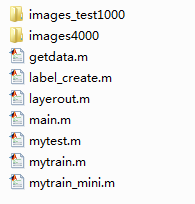运行main.m文件即可。
文件下载地址：链接：https://pan.baidu.com/s/1JhPs94qO-7VkPI_kknv_Tg 提取码：xuu6 如链接失效可加QQ:1021288218 索取。
参考： https://blog.csdn.net/yunyunyx/article/details/80473532
展开全文手写数字识别
• ## matlab元胞自动机入门详解

万次阅读 多人点赞 2019-02-09 11:13:08
胞自动机是定义在网格上的，每一个上的网格代表一个元胞与一种有限的状 态。变化规则适用于每一个元胞并且同时进行。 元胞的变化规则&amp;amp;amp;元胞状态 典型的变化规则，决定于元胞的状态，以及其（ 4 或 ...
元胞自动机的初步理解
对元胞自动机的初步认识 元胞自动机（CA）是一种用来仿真局部规则和局部联系的方法。典型的元 胞自动机是定义在网格上的，每一个点上的网格代表一个元胞与一种有限的状 态。变化规则适用于每一个元胞并且同时进行。元胞的变化规则&元胞状态 典型的变化规则，决定于元胞的状态，以及其（ 4 或 8 ）邻居的状态。元胞自动机的应用 元胞自动机已被应用于物理模拟，生物模拟等领域。元胞自动机的matlab编程 结合以上，我们可以理解元胞自动机仿真需要理解三点。一是元胞，在matlab中可以理解为矩阵中的一点或多点组成的方形块，一般我们用矩阵中的一点代表一个元胞。二是变化规则，元胞的变化规则决定元胞下一刻的状态。三是元胞的状态，元胞的状态是自定义的，通常是对立的状态，比如生物的存活状态或死亡状态，红灯或绿灯，该点有障碍物或者没有障碍物等等。
更新画面
动态的展示变化过程，需要掌握下面三条语句
h = imagesc(你的元胞矩阵)
在按照一定规则更行状态的循环中，
set(h,'cdata',你的更新后的元胞矩阵)
drawnow或者pause(时间间隔)

元胞自动机的有趣实例
生命游戏机
生命游戏是英国数学家约翰·何顿·康威在1970年发明的细胞自动机。它包括一个二维矩形世界，这个世界中的每个方格居住着一个活着的或死了的细胞。一个细胞在下一个时刻生死取决于相邻八个方格中活着的或死了的细胞的数量。通常情况，游戏的规则就是：当一个方格周围有2或3个活细胞时，方格中的活细胞在下一个时刻继续存活；即使这个时刻方格中没有活细胞，在下一个时刻也会“诞生”活细胞。 听说许多程序猿都喜欢玩这个 规则是： ¾ 对周围的 8 个近邻的元胞状态求和 ¾ 如果总和为 2 的话，则下一时刻的状态不改变 ¾ 如果总和为 3 ，则下一时刻的状态为 1 ¾ 否则状态= 0 元胞的邻居定义通常有以下三种范式，这里采用第二种，认为其周围八个点为邻居。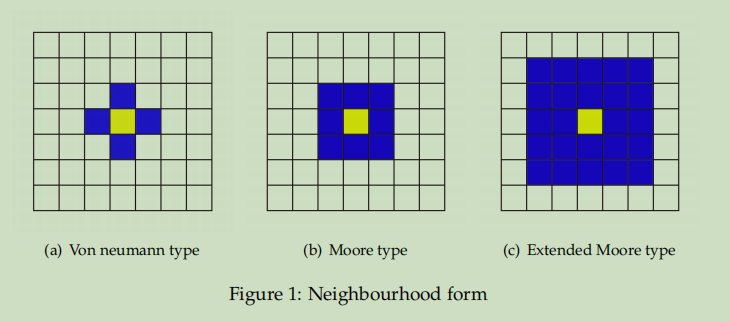简单仿真效果：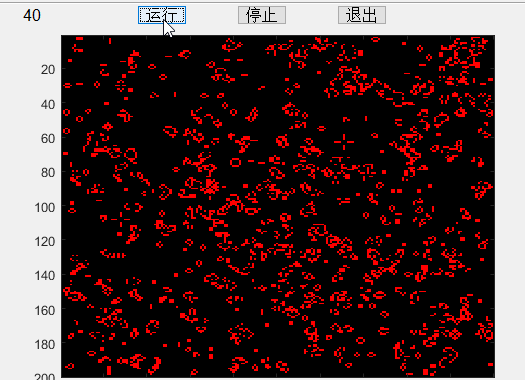全部代码如下
%% 设置GUI按键
plotbutton=uicontrol('style','pushbutton','string','运行', 'fontsize',12, 'position',[150,400,50,20], 'callback', 'run=1;');
erasebutton=uicontrol('style','pushbutton','string','停止','fontsize',12,'position',[250,400,50,20],'callback','freeze=1;');
quitbutton=uicontrol('style','pushbutton','string','退出','fontsize',12,'position',[350,400,50,20],'callback','stop=1;close;');
number = uicontrol('style','text','string','1','fontsize',12, 'position',[20,400,50,20]);
%% 元胞自动机设置
n=200;
%初始化各元胞状态
z = zeros(n,n);
sum = z;
cells = (rand(n,n))<.6;
% 建立图像句柄
imh = image(cat(3,cells,z,z));
set(imh, 'erasemode', 'none')
% 元胞更新的行列数设置
x = 2:n-1;
y = 2:n-1;
% 主事件循环
stop= 0; run = 0;freeze = 0;
while stop==0
if run==1
% 计算邻居存活的总数
sum(x,y) = cells(x,y-1) + cells(x,y+1) + cells(x-1, y) + cells(x+1,y)...
+ cells(x-1,y-1) + cells(x-1,y+1) + cells(x+1,y-1) + cells(x+1,y+1);
% 按照规则更新
cells = (sum==3) | (sum==2 & cells);
set(imh, 'cdata', cat(3,cells,z,z) )
stepnumber = 1 + str2double(get(number,'string'));
set(number,'string',num2str(stepnumber))
end
if freeze==1
run = 0;
freeze = 0;
end
drawnow
end

扩散
规则，先把中间点置为1，每一时间步对每一点，如果周围 八个点和为偶数，则变为0，为奇数则变为 1
效果：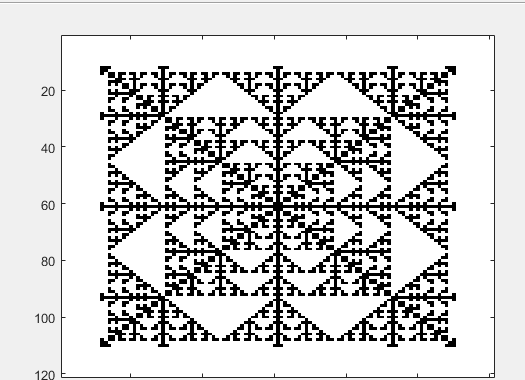代码：
% 颜色控制
Map = [1 1 1; 0 0 0];
colormap(Map);
% 设置网格大小
S = 121;
L = zeros(S);
% 把中间一个数设置为 1 作为元胞种子
M = (S+1)/2;
L(M, M) = 1;
Temp = L;
imagesc(L);
% 计算层数
Layer = (S-1)/2 + 1;

for t=2:Layer
for x=M-t+1:M+t-1
if x==M-t+1 || x==M+t-1

for y=M-t+1:M+t-1
SUM = 0;
for m=-1:1
for n=-1:1
if x+m>0 && x+m<=S && y+n>0 && y+n<=S
SUM = SUM + L(x+m, y+n);
end
end
end
SUM = SUM - L(x, y);
Temp(x, y) = mod(SUM, 2);
end

else
y = M-t+1;
SUM = 0;
for m=-1:1
for n=-1:1
if x+m>0 && x+m<=S && y+n>0 && y+n<=S
SUM = SUM + L(x+m, y+n);
end
end
end
SUM = SUM - L(x, y);
Temp(x, y) = mod(SUM, 2);

y = M+t-1;
SUM = 0;
for m=-1:1
for n=-1:1
if x+m>0 && x+m<=S && y+n>0 && y+n<=S
SUM = SUM + L(x+m, y+n);
end
end
end
SUM = SUM - L(x, y);
Temp(x, y) = mod(SUM, 2);
end
end
L = Temp;
imagesc(L);
% 速度控制
pause(0.2);
end

双车道交通流仿真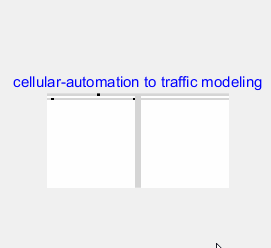这个稍微难一点
脚本
% 车流密度不变下的双向两车道仿真（T 字形路口）
% v:平均速度，d:换道次数（1000 次）p:车流密度
nl = 80 ;% 车道长度（偶数）
nc = 2; % nc:双向车道数目
dt= 0.01; % 仿真步长时间
fp = 20; % 车道入口处新进入车辆的概率（列向量）
nt=10000;% 仿真步长数目
chance=0.5;
chance1=0.5;
[ v, d, p ] = multi_driveway_with_crossroad_exit ( nl,nc,dt,fp,nt,chance,chance1);


规则&状态转移函数
function [ v, d, p ] = multi_driveway_with_crossroad_exit( nl,...
nc,dt,fp,nt,chance,chance1)
% fp:车道入口处新进入车辆的概率向量（2,3,5 车道）——输入参数
% chance:交叉口处车辆行为的概率向量(5 车道右转,3车道右转）——输入参数
%构造元胞矩阵
B=ones(nc+1+nl/2,nl+3);
%不可行车道
B(nc/2+1,[1:nl/2 nl/2+4:nl+3])=1.2;
B(nc+2:nc+1+nl/2,[1:nl/2 nl/2+4:nl+3])=1.2;
%初始化仿真元胞状态（1 为无车，0 为有车）
bb1=B([1:nc/2 nc/2+2:nc+1],:);bb2=B(:,nl/2+3);bb3=B(:,nl/2+1);
bb1(bb1~=0)=1;
bb2(bb2~=0)=1;
bb3(bb3~=0)=1;
B([1:nc/2 nc/2+2:nc+1],:)=bb1;B(:,nl/2+3)=bb2;B(:,nl/2+1)=...
bb3;B(1:nc+1,nl/2+1:nl/2+3)=1;
B(1:nc/2,end)=0;B(nc/2+2:nc+1,1)=0;B(end,nl/2+3)=0;
%显示初始交通流图
figure();
H=imshow(B,[]);
set(gcf,'position',[241 132 560 420]) ;%241 132 560 420
set(gcf,'doublebuffer','on'); %241
title('cellular-automation to traffic modeling','color','b');
%初始化化存储元胞上车辆状态的矩阵
S(1:nc*2+2,nl/2-2) = 0;
Q(1:nc*2+2,1:2) = 0;
C=zeros(nc+1,3);
%初始化换道频率、平均速度、车流密度相关变量
av(1:nt) = 0;
ap(1:nt) = 0;
s = 1;flag= 0;flag1=0;%flag、flag1 用于标示小区出口的车是否为左转车辆
flag2=0;
for n = 1:nt
%六个路段的长度。
A=[
B(1:nc/2,nl/2 :-1:1);
B(nc/2+2:nc+1,1:nl/2);
B(1:nc/2,nl+3:-1:nl/2+4);
B(nc/2+2:nc+1,nl/2+4:nl+3);
B(nc+1+nl/2:-1:nc+2,nl/2+3)';
B(nc+2:1:nc+1+nl/2,nl/2+1)'
];
c=B(1:nc+1,nl/2+1:nl/2+3);
%确定前 n-2 个车辆的状态
S(:,:) = 0;
S(A(:,1:end-2)==0&A(:,2:end-1)==1&A(:,3:end)==1)=2;%快速行驶的车
S(A(:,1:end-2)==0&A(:,2:end-1)==0)=3;%停车的车
S(A(:,1:end-2)==0&A(:,2:end-1)==1&A(:,3:end)==0)=1;%慢速行驶的车
%确定最后两个元胞的状态
Q(:,:)= 0;
Q(A(:,end-1)==0&A(:,end)==0) = 3;
Q(A(:,end-1)==0&A(:,end)==1) = 1;
if c(3,1)==0
if rand<chance1
flag2=1;
c(3,1)=1;
end
end
if A(1,end)==0
Q(1,end)=1;
end
if A(4,end)==0
Q(4,end)=1;
end
if A(6,end)==0
Q(6,end)=1;
end
if rem(floor(n/50),2)==0 %此时左右向为绿灯
if A(2,end)==0
if c(nc/2+2:nc+1,1)==0
Q(2,end)=3;
else
Q(2,end)=1;
end
end
if A(3,end)==0
if c(1,3)==0
Q(3,end)=3;
else
Q(3,end)=1;
end
end
%按照既定规则行驶（5 车道右转）
if A(5,end)==0
if flag==0
if rand<chance %路口车右转
if c(nc/2+2:nc+1,:)==1
Q(5,end)=1;
else
Q(5,end)=3;
end
end
else %第一辆车为左转车，需要等待
end
end
if c(1,2)==0
if c(1,1)==1%3道口左转的思路：规避。
C(1,2)=1;
else
C(1,2)=3;
end
if c(2,1)==0
C(1,2)=3;
end
end
if c(1,3)==0
if c(1,2)==1
C(1,3)=1;
else
C(1,3)=3;
end
end
if c(3,1)==0
if c(3,2)==1
C(3,1)=1;
else
C(3,1)=3;
end
end
if c(3,2)==0
if c(3,3)==1
C(3,2)=1;
else
C(3,2)=3;
end
end
if rem(n,20)==0&&c(3,2)==0%小区出来的车还遗留在路口，特殊处理先行
if c(2,1)==1
C(3,2)=5; %特殊的等待状态（小区出来的车）
else
C(3,2)=3;
end
end
if c(2,1)==0
if A(1:nc/2,1)==0
C(2,1)=3;
else
C(2,1)=1;
end
end
if c(1,1)==0
if A(1,1)==0
C(1,1) = 3;
else
C(1,1) = 1;
end
end
if c(3,3)==0
if A(nc*3/2+1:2*nc,1)==0
C(3,3) = 3;
else
C(3,3) = 1;
end
end
else %此时小区出入向为绿灯
Q(2,end)=3;Q(3,end)=3;
if c(3,2)==0
if flag1==1
if c(2,1)==1
C(3,2)=5;flag1=0;
else
C(3,2)=3;
end
else
if c(3,3)==1
C(3,2)=1;
else
C(3,2)=3;
end
end
end
if c(2,1)==0
if A(1:nc/2,1)==1&&c(1,1)==1
C(2,1)=1;
else
C(2,1)=3;
end
end
if A(5,end)==0
if flag==0
if rand<chance
if c(nc/2+2:nc+1,:)==1
Q(5,end)=1;
else
Q(5,end)=3;
end
else
if c(nc/2+2:nc+1,1)==1&&c(nc/2+2:nc+1,2)==1
Q(5,end)=5;flag=0;flag1=1; %小区的左转前进，用以区分右转车辆
else
Q(5,end)=3;flag=1;
end
end
else
if c(nc/2+2:nc+1,1)==1&&c(nc/2+2:nc+1,2)==1
Q(5,end)=5;flag=0;flag1=1; %小区的左转前进，用以区分右转车辆
else
Q(5,end)=3;flag=1;
end
end
end
if c(1,2)==0
if c(1,1)==1
C(1,2)=1;
else
C(1,2)=3;
end
end
if c(1,3)==0
if c(1,2)==1
C(1,3)=1;
else
C(1,3)=3;
end
end
if c(3,1)==0
if c(3,2)==1
C(3,1)=1;
else
C(3,1)=3;
end
end
if c(1,1)==0
if A(1:nc/2,1)==0
C(1,1) = 3;
else
C(1,1) = 1;
end
end
if c(3,3)==0
if A(nc*3/2+1:2*nc,1)==0
C(3,3) = 3;
else
C(3,3) = 1;
end
end
end
%获得所有元胞上车辆的状态
Acc = [ S Q ];
%根据当前状态改变元胞位置
%路口附近的车辆的行驶控制
if C(3,2)==5
c(2,1)=0;
c(3,2)=1;
flag=0;
C(3,2)=0;
elseif C(3,2)==1
c(3,3)=0;
c(3,2)=1;
C(3,2)=0;
end
if C(2,1)==1
A(1,1)=0;
c(2,1)=1;
C(2,1)=0;
end
if Acc(3,end)==1
c(1,3)=0;
A(3,end)=1;
Acc(3,end)=0;
end
if Acc(2,end)==1
c(3,1)=0;
A(2,end)=1;
Acc(2,end)=0;
end
if C(3,1)==1
c(3,2)=0;
c(3,1)=1;
C(3,1)=0;
end
if C(1,3)==1
c(1,2)=0;
c(1,3)=1;
C(1,3)=0;
end
if C(1,2)==1
c(1,1)=0;
c(1,2)=1;
C(1,2)=0;
end
if C(1,1)==1
A(1,1)=0;
c(1,1)=1;
C(1,1)=0;
end
if C(3,3)==1
A(4,1)=0;
c(3,3)=1;
C(3,3)=0;
end
%慢速运行车辆向前走 1 格
A( Acc(:,1:end)==1 )=1;
A( [ zeros(nc*3,1) Acc(:,1:end-1)]==1 ) = 0;
%高速运行车辆向前走 2 格
A( Acc(:,1:end)==2) = 1;
A( [ zeros(nc*3,2) Acc(:,1:end-2)]==2) = 0;
if Acc(1,1)==1||Acc(1,1)==2
A(1,1)=1;
end
if Acc(4,1)==1||Acc(4,1)==2
A(4,1)=1;
end
if Acc(5,end)==5
c(3,2)=0;flag=0;
A(5,end)=1;
elseif Acc(5,end)==1
c(3,3)=0;
A(5,end)=1;
end
if Acc(3,end)==1
c(1,3)=0;
A(3,end)=1;
end
if Acc(2,end)==1
c(3,1)=0;
A(2,end)=1;
end
if Acc(4,1)==1||Acc(4,1)==2
A(4,1)=1;
end
if Acc(1,1)==1||Acc(1,1)==2
A(1,1)=1;
end
%计算平均速度、换道频率、车流密度等参数
%获得运行中的车辆数目 N
matN = A<1;
N = sum(sum(matN));
%获得运行中的车辆速度之和 V
E = S((S==1)|(S==2));
V = sum(E);
%计算此时刻的车流密度并保存
ap(n) = N/( (nc*3)*(nl/2)+9 );
%计算此时刻的平均速率并保存
if(N~=0&&n>nl/2)
av(s) = V/N;
s = s+1;
end
%在车道入口处随机引入新的车辆
A([2;3;5],1)=(round(fp.*rand(3,1))&A([2;3;5],1));
A(A~=0)=1;
if flag2==1
A(6,1)=0;
flag2=0;
end
%将新的车辆加入元胞矩阵中
B(1,1:nl/2)=A(1:nc/2,end:-1:1);
B(3,1:nl/2)=A(nc/2+1:nc,:);
B(1,nl/2+4:nl+3)=A(nc+1:nc*3/2,end:-1:1);
B(3,nl/2+4:nl+3)=A(nc*3/2+1:2*nc,:);
B(nc+2:nc+1+nl/2,nl/2+3)=A(2*nc+1,end:-1:1)';
B(nc+2:nc+1+nl/2,nl/2+1)=A(3*nc,:)';
B(1:3,nl/2+1:nl/2+3)=c(:,:);
%显示交通流图
set(H,'CData',B);
%计算这个时间段每个时间点的指标（速度与车流量）。
p = mean(ap);
v = sum(av)/s;
disp([v,p])
%仿真步长
pause(dt);
end
end

展开全文元胞自动机
• ## MATLAB添加噪声

万次阅读 2019-05-23 20:54:02
首先是用MATLAB自带的imnoise函数添加噪声。
img= im2double(imread('D:\Matlab2018a\work\img.png'));%改为你要读入图片的路径;im2double作归一化处理
sigma=25;%添加噪声的标准差
imgnoise=imnoise(img,'gaussian',0,(sigma/255)^2); %添加均值为0，标准差为sigma高斯噪声
imshow(imgnoise);
imwrite(imgnoise,'imgnoise.png');%输出噪声图到当前文件夹

由于matlab的imnoise函数会将输出归一化，所以在读入图片的时候就先用im2double把图片归一化了，免得数据类型不一样。 将上面添加噪声那一句改为如下语句则添加其它种类的噪声
imgnoise=imnoise(img,'salt & pepper',0.05); %添加密度为5%的椒盐噪声
imgnoise=imnoise(img,'speckle',(sigma/255)^2); %添加均值为0，标准差为sigma乘性噪声，这个是用服从均匀分布的随机噪声乘以图像img，再加到img上。
imgnoise=imnoise(img,'poisson');%添加泊松噪声

下面我们用自己写的代码来添加噪声。
img=img+(sigma/255)*randn(size(img));%给img添加标准差为sigma的高斯噪声
img=img+img.*((sigma/255)*rand(size(img)));%给img添加标准差为sigma的乘性噪声，也是均匀分布

对于椒盐噪声，先用rand()生成均匀分布的随机噪声，然后根据你需要的椒盐噪声密度，作阈值处理，反正就是这种思想，实现的方法有很多，我这里列一种。
img=im2double(imread('8.png'));%改为你要读入图片的路径
imgnoise =(rand(size(img))); %生成随机数组
d = 0.1;  %需要的椒盐噪声密度
output=img;
[width,height]=size(img);

for i=1:width
for j=1:height
if(imgnoise(i,j)>(1-d/2))
output(i,j)=1;
end
if(imgnoise(i,j)<d/2)
output(i,j)=0;
end
end
end
imshow(output);

也可以分两步分别生成椒噪声和盐噪声，但是这个总的密度就没有那么好控制
img=im2double(imread('8.png'));%改为你要读入图片的路径
d = 0.1;  %需要的胡椒或盐粒噪声密度
output=img;
[width,height]=size(img);
a1=rand(width,height)< d^0.5;
a2=rand(width,height)< d^0.5;
output(a1&a2) = 1; %生成盐噪声
b1=rand(width,height)< d^0.5;
b2=rand(width,height)< d^0.5;
output(b1&b2) = 0; %生成胡椒噪声
imshow(output);
%椒盐噪声的密度就是d+d-d*d

展开全文添加噪声 椒盐噪声
• 包含加密m文件，解密m文件，两个函数，都已搞好，数字内容安全作业
• ## matlab awgn函数加入高斯白噪声

千次阅读 多人点赞 2020-09-15 21:36:23
标量snr指定了每一个采样信号与噪声的比率，单位为dB。如果x是复数的，awgn将会添加复数噪声。这个语法假设x的能量是0dBW。 y = awgn(x,snr,sigpower)和上面的语法相同，除了sigpower是x的能量，单位为dBW。 y = ...
信噪比
信噪比，电子设备或者通信系统中有效信号和噪声的比值，英文名称叫做SNR或S/N（SIGNAL-NOISE RATIO)；
awgn描述
awgn函数可以将白色高斯噪声添加到信号中。
语法
y = awgn(x,snr)
y = awgn(x,snr,sigpower)
y = awgn(x,snr,'measured')
y = awgn(x,snr,sigpower,state)
y = awgn(x,snr,'measured',state)
y = awgn(...,powertype)

描述
y = awgn(x,snr)将白高斯噪声添加到向量信号x中。标量snr指定了每一个采样点信号与噪声的比率，单位为dB。如果x是复数的，awgn将会添加复数噪声。这个语法假设x的能量是0dBW。y = awgn(x,snr,sigpower)和上面的语法相同，除了sigpower是x的能量，单位为dBW。y = awgn(x,snr,‘measured’)和y = awgn(x,snr)是相同的，除了agwn在添加噪声之前测量了x的能量。y = awgn(x,snr,sigpower,state)和y = awgn(x,snr,sigpower)是相同的，除了awgn首先重置了正态随机数产生器randn的状态为整数状态。y = awgn(x,snr,‘measured’,state)和y = awgn(x,snr,‘measured’)是相同的，除了awgn首先重置了正态随机数产生器randn的状态为整数状态。y = awgn(…,powertype)和前面的语法相同，除了字符串powertype指定了snr和sigpower的单位。powertype的选择有’db’ and ‘linear’，如果powertype是’db’，那么snr是按照dB为单位测量的，sigpower是按照dBW为单位测量的。如果powertype是线性的，snr是按照一个比率测量的，sigpower是以瓦特为单位测量的。Relationship Among SNR, Es/N0, and Eb/N0 对于SNR和其他的噪声相对能量测量的关系，查看Describing the Noise Level of an AWGN Channel。
例子
%	Example 1:
% To specify the power of X to be 0 dBW and add noise to produce
% an SNR of 10dB, use:
X = sqrt(2)*sin(0:pi/8:6*pi);
Y = awgn(X,10,0);

%	Example 2:
% To specify the power of X to be 3 Watts and add noise to
% produce a linear SNR of 4, use:
X = sqrt(2)*sin(0:pi/8:6*pi);
Y = awgn(X,4,3,'linear');

%   Example 3:
% To cause awgn to measure the power of X and add noise to
% produce a linear SNR of 4, use:
X = sqrt(2)*sin(0:pi/8:6*pi);
Y = awgn(X,4,'measured','linear');

%   Example 4:
% To specify the power of X to be 0 dBW, add noise to produce
% an SNR of 10dB, and utilize a local random stream, use:
S = RandStream('mt19937ar','seed',5489);
X = sqrt(2)*sin(0:pi/8:6*pi);
Y = awgn(X,10,0,S);

%    Example 5:
% To specify the power of X to be 0 dBW, add noise to produce
% an SNR of 10dB, and produce reproducible results, use:
reset(RandStream.getGlobalStream)
X = sqrt(2)*sin(0:pi/8:6*pi);
Y = awgn(X,10,0,S);

matlab 程序 正弦+白噪声

f1=50;  	%  频率
fs=1000;  	%  采样频率
Ts=1/fs;  	%  采样间隔
N=200;    	%  采样点数
n=1:N;
y=sin(2*pi*f1*n*Ts);
snr=30;		%  信噪比
r=awgn(y,snr);
subplot(2,1,1);
plot(y,'black')
axis([-inf,inf,-2,2,])
title('原信号');
subplot(2,1,2);
%hold on
plot(r,'black')
axis([-inf,inf,-2,2,])
title('原信号+高斯噪声');


SNR=10SNR=20SNR=30matlab 程序 锯齿波+噪声
t = 0:.1:20;
x = sawtooth(t); % Create sawtooth signal.
snr=10;

y = awgn(x,snr,'measured'); % Add white Gaussian noise.
subplot(1,3,1);
plot(t,x,t,y) % Plot both signals.
legend('Original signal','Signal with AWGN');
axis([-inf,inf,-1.5,1.5,])
title('SNR=10');

snr=20;
y = awgn(x,snr,'measured'); % Add white Gaussian noise.
subplot(1,3,2);
plot(t,x,t,y) % Plot both signals.
legend('Original signal','Signal with AWGN');
axis([-inf,inf,-1.5,1.5,])
title('SNR=20');

snr=30;
y = awgn(x,snr,'measured'); % Add white Gaussian noise.
subplot(1,3,3);
plot(t,x,t,y) % Plot both signals.
legend('Original signal','Signal with AWGN');
axis([-inf,inf,-1.5,1.5,])
title('SNR=30');

修改信噪比展开全文awgn
• ## 路径规划A*算法matlab代码注释

万次阅读 多人点赞 2019-01-17 21:29:31
路径规划A*算法matlab代码注释 文中所使用的代码出自手把手教用matlab做无人驾驶（三）-路径规划A*算法 一、Aplanning.m disp('A Star Path Planing start!!') p.start=[1,1]; %起始 p.goal=[3,5]; %目标 ...路径规划 A*算法
• ## matlab 实现透视变换 倾斜校正

千次阅读 热门讨论 2019-09-08 20:23:01
说明：输入：四个的坐标，输出：想要的矩形的大小。也就是对任意四边形都可以经过透视变换进行校正成你想要的矩形。（因为我处理的是视频，所以里面有循环进行校正的代码） 这是循环的处理视频的每一帧，对每一帧...
• Matlab模糊控制工具箱为模糊控制器的设计提供了一种非常便捷的途径，通过它我们不需要进行复杂的模糊化、模糊推理及反模糊化运算，只需要设定相应参数，就可以很快得到我们所需要的控制器，而且修改也非常方便。...模糊控制工具箱 模糊控制pid
• %加入椒盐噪声 Hsp=imnoise(I,'salt & pepper'); subplot(2,2,2); imshow(Hsp); title("椒盐噪声图像"); %用均值滤波器去除图像中的噪声 Kaverage = imfilter(Hsp,fspecial('average',3)); subplot(2,2,3); ...图像识别 计算机视觉
• 加入车辆行驶路径，并加入一台camera 导出到SIMULINK，自动生成slx与mat文件 SCENARIO模块输出的车辆数据格式如下，可用Bus模块分解，也可作为结构体使用matlab function 解码 1.2 使用现有场景 如图所示，...
• ## matlab 滤波器篇

千次阅读 多人点赞 2016-02-19 23:15:47
快毕业了，把自己写的现成的matlab函数分享给有需要的人，由于个人水平有限，写的不好请见谅，愿意拍砖的尽管拍好了。目前还不考虑读博，所以写的程序仍了可惜，所以就拿出来分享。好了不废话了，开始正题。   ...
• ## 基于MATLAB的水果分级设计

万次阅读 多人点赞 2018-06-14 14:19:35
基于MATLAB的水果分级识别技术研究摘 要本次毕业设计介绍了基于MATLAB的水果分级自动识别，利用手机端获取苹果的样本图像，应用MATLAB软件编程实现了对样本图像的预处理，包括图像滤波、图像填充、图像灰度化、图像...
• matlab 代码关于 批量加入legend 以及修改 xlabel ylabel 字体 （宋体 以及 TIMES NEW ROMAN）
• ## 蚁群算法---matlab代码

万次阅读 多人点赞 2017-08-03 00:39:50
蚁群算法—matlab代码文明转帖，代码摘自：FPGA机器学习之蚁群算法 matlab程序蚁群算法简介 蚁群是自然界中常见的一种生物，人们对蚂蚁的关注大都是因为“蚁群搬家，天要下雨”之类的民谚。然而随着近代仿生学的...机器学习 蚁群算法
• ## 基于MATLAB的人脸识别算法的研究

万次阅读 多人点赞 2017-12-04 20:24:32
基于MATLAB的人脸识别算法的研究 作者：lee神 现如今机器视觉越来越盛行，从智能交通系统的车辆识别，车牌识别到交通标牌的识别；从智能手机的人脸识别的性别识别；如今无人驾驶汽车更是应用了大量的机器识别的是...脸部识别 图像处理 算法 机器视觉
• 一份白皮书，介绍了使用 VB 代码（在示例 Master.bas 文件中提供）调用任何 MATLAB 代码或从 PowerPoint 中运行任何 MATLAB 文件的简单工作流程。 只需按照说明编辑 .bas 文件，然后将其加载到您的 PowerPoint 中。 ...
• 这是如何在 MATLAB:registered: 中向绘图添加文本的示例。 阅读 MATLAB 文档中的“文本”函数。 有关更多示例，请转到 MATLAB 绘图库 - http://www.mathworks.com/discovery/gallery.html
• 另外，需要声明的是，我所使用的MATLAB版本——MATLAB R2017a。 目录 一、使用MATLAB的GUIDE开发环境，来快速创建GUI界面 二、修改MATLAB GUI的.fig和.m文件名 三、修改MATLAB GUI界面的名字 四...
• ## MATLAB中调用eemd函数

万次阅读 多人点赞 2019-07-16 15:18:06
#MATLAB 中调用EEMD 函数 一般情况添加eemd.m和extrema.m到主函数的同一个文件夹就可直接调用了。 eemd.m中是英文注释，解释该函数各个参量的意义以及如何取值。 function allmode=eemd(Y,Nstd,NE) Y为输入，待分解...
• matlab编程实现图像加入噪声去除噪声，加入各种噪声，包括椒盐和高斯噪声。
• 添加高斯白噪声的MATLAB代码，高斯白噪声经常被作为噪声被添加在图片等上，作为水印
• 加入噪声时用注释中的y公式。 clc % 时域图 f = 10 T = 1 fs = 100 n = T*fs t = linspace(0,T,n) % 定义的原始无噪声的信号 y = 2*cos(2*pi*f*t+pi/6) % 如ALL-DIGITAL FREQUENCY SYNTHESIZER IN DEEP-SUBMICRON ...
• ## matlab按点绘制网格

千次阅读 2019-12-08 15:07:59
为了看图的简洁，我想让matlab画图时，在坐标处分别绘制水平和竖直的参考线，由此引出了下面的内容。 首先我们要了解的是set函数。 matlab中的set函数 MATLAB给每种对象的每一个属性规定了一个名字，称为属性名，...
• 由于BP神经网络的收敛速度慢，不适合安在自适应系统里，所以选择了速度比较快的RBF神经网络，看了关于RBF神经网络自适应控制的一些原理和MATLAB代码，有一些自己的理解写在这里。 一般的神经网络的作用是去做一些...
• ## MATLAB--数字图像处理 添加高斯噪声

万次阅读 多人点赞 2019-09-14 17:03:02
subplot(1,2,2),imshow(t1),title('加入均值为0，标准差为0.1的高斯噪声后'); 效果图： 方法二 ：随机生成高斯噪声(这个我还没有懂那个随机生成高斯噪声函数怎么来的!) 代码： image=imread('a1.jpg'); ...数字图像处理 添加高斯噪声
• matlab开发-添加删除列表。将元素从一个列表添加到另一个列表并将其删除。可以多次添加/删除。
• Matlab如何在figure画图legend中加入分数latex代码？.docx...

# matlab加入点matlab 订阅